## Thursday, June 01, 2006

### Growing Post

Question 1: What is the difference between an algerbraic expression and an equation (Hint: One contains this and the other doesn't)? Give an example of both an expression and an equation.

An Algebraic expression is when you combine numbers, operatives, and/or free variables and bound variables. You get to choose the variable in an algebraic expression. An example: 5A X 6.
In Algebraic equation, the variable is already given. There are two types of equations (from my knowledge), one step; 3E - 6 = 9, and two step; 3N = 1N +10. An example of an equation; 3H - 5 = 10. H = 5.

Question 2: What is a variable and why do we use one in algebra?

Variables are letters that represent the unknown, able to change. Variables are often contrasted with constants, which are known and unchangning.
We use variables because Algebra is a method of finding the unknown.

Question 3: Solve for N

To solve the first question, you need to figure out what a triangle equals on the first scale. First, take away four circles (each square equals two circles), which leaves five circles. Since there were five circles left, the triangle equals five circles.
To solve the second question, you need to figure out the value of the two N's.
First, add the squares and triangles together. The total of the squares and triangles, together, was 22 circles. Then divide the 22 circles by 2. The answer was 11. Each N equals 11 circles.

Question 4: Solve the following equations. Show all of the steps that are needed.

A) 3n+4n+7=2n+12

3N + 4N - 2N = 12 - 7
5N = 5
N=1

B) 8n-(4+9)=11

8N = 13 + 11

13 + 11 = 24

8N = 24

N = 3

C) (8-3)n+7n+8= 4n + 40

5N + 7N + 8= 4N + 40
5N + 7N - 4N = 40 - 8

8N = 32

N = 4

Question 5: For this question you need to create a T-Chart and a graph to plot your data from this question onto.
You are having a race against your friend, except you are on foot and he is on his bike. Your both know that if you are on a bike you will be faster so your friend gives you a head start of 3 minutes. If you can run 400m per minute, and your friend can bike 700m per minute, who will be the first to make it to the
finish line 2000m away?
The runner will finish in five minutes, the bicyclist will finish in six minutes, therefore the runner winning.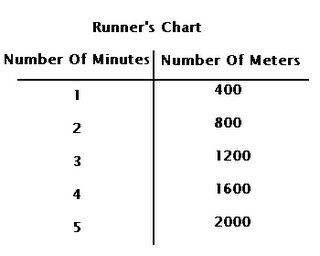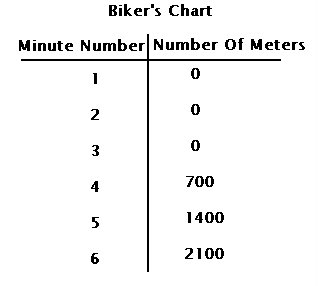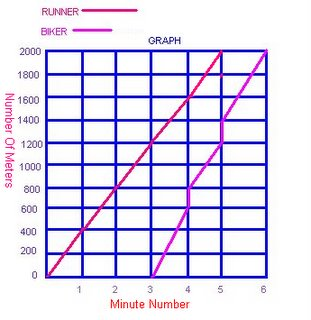## Monday, May 29, 2006

### Kim's growing Post for May 29

Question 1.
What is the difference between an algebraic expression and an equation (hint:one contains this and the other other doesn't?)

The difference bewteen an algebraic expression and an equation is that the algebraic expression has not given the value of the variable and the equation has already given us the value of the variable. (example:)
expression: 10 + 5n = the answer
equation: 10 + 5(3) =
45

Question 2.
What is a variable and why do we use one in algebra.

A variable represents the unknown number. We use a variable in algebra because the main thing about algebra is to find the unknown answer and since the variable is the unknown number, we have to solve the variable. (And if you have solved the variable, it makes it a whole lot easier to solve the equation.)

Question 3.
Solve for N.

I need to solve how much the triangular equals to solve the question. After that, I need to solve how much the 2 N's equal to.
Solving for first scale: Since a square means 2 circles and a circle means 1, and the left side of the scale in this diagram has 2 squares, that means 4 circles or 4 in all. So I take 4 circles away from the right hand of the scale, I will end up having only 5 circles left and a triangle. So that means a triangle is equal to 5 circles.
Solving for second scale: Since I have already solved the value of the triangle and I know what the value of the circle and the square is, I will be able to solve the value of the 2 N's easily. First I would have to add all the squares and triangles on the right hand of the scale, and it would come out of a total of 22 circles or 22 (since a triangle represents 5 circles and a square represents 2 circles.) Now I will have to divide the number 22 in half (because there are 2 Ns) and I will get 11. So the value of each N will be 11. The total value of both Ns is 22 circles.

Question 4.
Solve the following equations. Show all of the steps that are needed.

a) 3n + 4n + 7 = 2n + 12
3n + 4n - 2n = 12 - 7
5n = 5
n = 5/5 = 1
n = 1

b) 8n - (4 + 9) = 11
8n - 13 = 11
8n = 11 + 13 = 24
8n = 24
n = 24/8 = 3
n = 3

c) (8-3)n + 7n + 8 = 4n + 40
5n + 7n + 8 = 4n + 40
5n + 7n - 4n = 40 - 8
8n = 32
n = 32/8 = 4
n = 4

Question 5. For this question you need to create a T-chart and a graph to plot your data from this question onto.
You are having a race against your friend, except you are on foot and he is on his bike. You both know that if you are on bike you will be faster so your friend gives you a head start of 3 minutes. If you can run 400m per minute, and your friend can bike 700m per minute, who will be the first one to make it to the finish line 2000m away?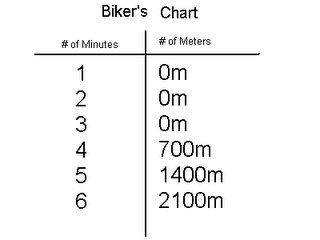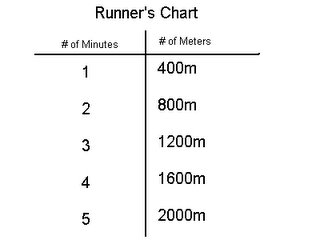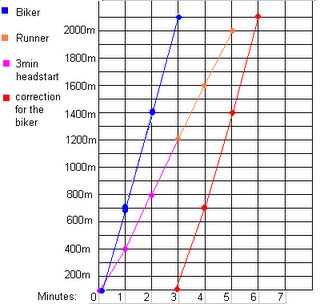The runner will come in first in 5 minutes and the biker will come in second in about 6 minutes.

## Sunday, May 28, 2006

### Janna's Growing Post for May 29th 2006

Question 1: What is the difference between an algerbraic expression and an equation (Hint: One contains this and the other doesn't)? Give an example of both an expression and an equation.

An algebraic expression is when you combine numbers, operatives, grouping symbols (such as brackets and parentheses) and/or free variables and bound variables. You get to choose the variable in an algebraic expression. An example of an algebraic expression is 20J / 5. In an algebraic equation is when you combine numbers, operatives, grouping symbols (such as brakets and parentheses) and/or free variables and bouund variables. In an equation the value of the variable is given. A example for an algebraic equation is 20J / 5 = 20. J equals 5.

Question 2: What is a variable and why do we use one in algebra?

A variable is a letter that replaces a number we are solving for. The variable stands for the unknown. We use a variable (a letter) in algebra so the equations we are solving do not become confusing. Example is we use numbers as variables instead of letters.
21 + 13 = 17 This equation doesn't make sense. Now here's the same equation except we use letters for the variable. 2A + 13 = 17. A eaquals 2. You multiply 2 by A which is 2. 2 x 2= 4. Your final equation would be 4 + 13 = 17.

Question 3: Solve for N.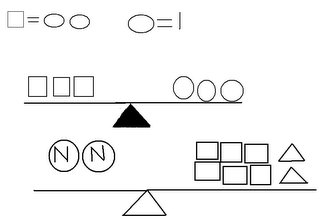I found that since 2 circles equals 1 square and a circle equals one, that a square equals 2. To solve the second question you need to figure out what a triangle equals on the first scale. I took away four circles (each square equals two circles), and it left me with five circles. Since there were five circles left the triangle equals five circles each. Then I needed to solve the N's. If each square equals 2 and each triangle equals f circles each which is 10. I added it up and got 22. that means you now have to divide 22 in half and you'd get 11. So N eqauls 11.

Question 4: Solve the following equations. Show all of the steps that are needed.

a.) 3n+4n+7=2n+12
7n+7=2n+127n-2n(+7-7)=2n-2n(+12-7)
5n=5
N=1

b) 8n-(4+9)=1
1 8(3)-(4+9)=
1124-13=11
N= 3

(c) (8-3)n+7n+8=
4n+405n+7n+8=
4n+405(4)+7(4)+8=
4(4)+4020+28+8=56
N= 4

Question 5: For this question you need to create a T-Chart and a graph to plot your data from this question onto.

You are having a race against your friend, except you are on foot and he is on his bike. Your both know that if you are on a bike you will be faster so your friend gives you a head start of 3 minutes. If you can run 400m per minute, and your friend can bike 700m per minute, who will be the first to make it to the finish line 2000m away?The runner will cross the finish line first in 5 minutes. The biker will cross the finish line second in 6 minutes.

### My Growing Post

1. In an algebraic expression you get to choose the value of the variable.
In an algebraic equation the value of the variable is given.

2.A variable is a letter that replaces a number. We use a variable in algebra because algebra is about solving the uknown and the variable is the unknown thing we have to solve.

3.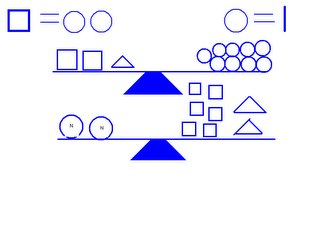To solve the second question you need to figure out what a triangle equals on the first scale. First I took away four circles (each square equals two circles), and it left me with five circles. Since there were five circles left the triangle equals five circles. To solve the second question you need to figure out the value of the two Ns. First I added the squares and triangles together. The total of the squares and triangles and it was 22 circles. Then I divided the 22 circles by 2. The answer was 11. So each N equals 11 circles.

4. .(a) 3n+4n+7=
2n+123(1)+4(1)+7=
2(1)+123+4+7=14
N= 1

b) 8n-(4+9)=1
1 8(3)-(4+9)=
1124-13=
11N= 3

(c) (8-3)n+7n+8=
4n+405n+7n+8=
4n+405(4)+7(4)+8=
4(4)+4020+28+8=56
N= 4

5.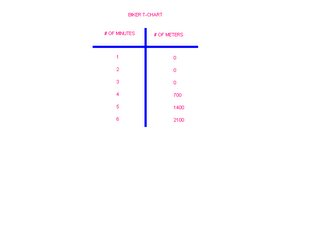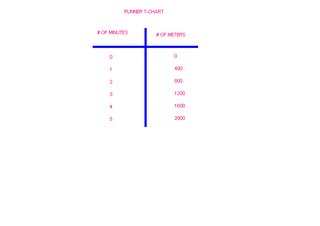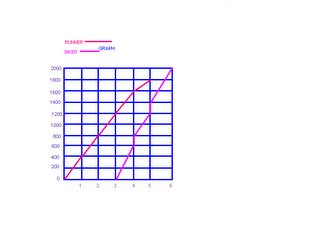The Runner will cross the finish line first in 5 minutes. The biker will cross the finish line second in 6 minutes.

### GROWING POSSTT !!

Question 1.) What is the difference between an algebraic expression and an equation (hint: One contains this and the other doesn't)? Give an example of both an expression and an equation.

Algebraic Expression you get to choose the value of the variable.
Algebraic Equation the value of the variable is already given.
Algebraic Expression: 8A+6
Algebraic Equation: 8A+6=30

Question 2.) What is a variable and why do we use one in algebra?

A variable is a letter that replaces a number. We use it in algebra beacause algebra is about finding the unknown, and the variable is the unknown thing we must solve in a problem.

Question 4.) Solve the following equations. Show all of the steps that are needed.

a.) 3n+4n+7=2n+12
7n+7=2n+127n-2n(+7-7)=2n-2n(+12-7)
5n=5
n=1

b.) 8n-(4+9)=11
8n-13=11
+13 +13
8n=24
n=24/8
n=3

c.) (8-3)n+7n+8=4n+40
5n+7n+8 =4n+40
12n+8=4n+40
12n(+8-8)=4n(+40-8)
12n-4n=(4n-4n)+32
8n=32
n=4

Question 5.) For this question you need to create a T-Chart and a graph to plot your data from this question onto.

You are having a race against your friend, except you are on foot and he is on his bike. Your both know that if you are on a bike you will be faster so your friend gives you a head start of 3 minutes. If you can run 400m per minute, and your friend can bike 700m per minute, who will be the first to make it to the finish line 2000m away?

The runner will cross the finish line first in 5 minutes. The biker will cross the finish line second in 6 minutes.